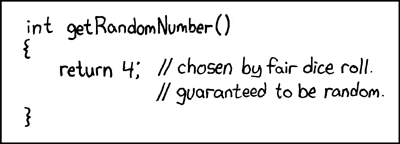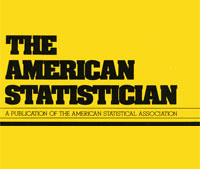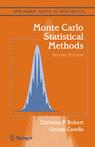biased sample!

Posted in Statistics with tags , , , , , , , , , , , on May 21, 2019 by xi'anA chance occurrence led me to this thread on R-devel about R sample function generating a bias by taking the integer part of the continuous uniform generator… And then to the note by Kellie Ottoboni and Philip Stark analysing the reason, namely the fact that R uniform [0,1) pseudo-random generator is not perfectly continuously uniform but discrete, by the nature of numbers on a computer. Knuth (1997) showed that in this case the range of probabilities is larger than (1,1), the largest range being (1,1.03). As noted in the note, exploiting directly the pseudo-random bits of the pseudo-random generator. Shocking, isn’t it!  A fast and bias-free alternative suggested by Lemire is available as `dqsample::sample`

Fisher’s lost information

Posted in Books, Kids, pictures, Statistics, Travel with tags , , , , , , , on February 11, 2019 by xi'anAfter a post on X validated and a good discussion at work, I came to the conclusion [after many years of sweeping the puzzle under the carpet] that the (a?) Fisher information obtained for the Uniform distribution U(0,θ) as θ⁻¹ is meaningless. Indeed, there are many arguments:

1. The lack of derivability of the indicator function for x=θ is a non-issue since the derivative is defined almost everywhere.
2. In many textbooks, the Fisher information θ⁻² is derived from the Fréchet-Darmois-Cramèr-Rao inequality, which does not apply for the Uniform U(0,θ) distribution.
3. One connected argument for the expression of the Fisher information as the expectation of the squared score is that it is the variance of the score, since its expectation is zero. Except that it is not zero for the Uniform U(0,θ) distribution.
4. For the same reason, the opposite of the second derivative of the log-likelihood is not equal to the expectation of the squared score. It is actually -θ⁻²!
5. Looking at the Taylor expansion justification of the (observed) Fisher information, expanding the log-likelihood around the maximum likelihood estimator does not work since the maximum likelihood estimator does not cancel the score.
6. When computing the Fisher information for an n-sample rather than a 1-sample, the information is n²θ⁻², rather than nθ⁻².
7. Since the speed of convergence of the maximum likelihood estimator is of order n⁻², the central limit theorem does not apply and the limiting variance of the maximum likelihood estimator is not the Fisher information.

uniform on the sphere [or not]

Posted in pictures, R, Statistics with tags , , , , , , , , , , , , on March 8, 2018 by xi'anWhile looking at X validated questions, I came upon this comment that simulating a uniform distribution on a d-dimensional unit sphere does not proceed from generating angles at random on (0,2π) and computing spherical coordinates… Which I must confess would have been my initial suggestion! This is obvious, nonetheless, when computing the Jacobian of the spherical coordinate transform, which involves powers of the sines of the angles, in a decreasing sequence from d-1 to zero. This means that the angles should be simulated according to their respective sine-power densities. However, except for the d=3 case, where simulating from the density sin(φ) is straightforward by inverse cdf, i.e. φ=acos(1-2u), the cdfs for the higher powers are combinations of sines and cosines, and as such are not easily inverted. Take for instance the eighth power:

F⁸(φ)=(840 φ – 672 sin(2 φ) + 168 sin(4 φ) – 32 sin(6 φ) + 3 sin(8 φ))/3072

While the densities are bounded by sin(φ), up to a constant, and hence an accept-reject can be easily derived, the efficiency decreases with the dimension according to the respective ratio of the Wallis’ integrals, unsurprisingly. A quick check for d=4 shows that the Normal simulation+projection-by-division-by-its-norm is faster.Puzzling a bit further about this while running, I wondered at the simultaneous simulations from sin(φ), sin(φ)², sin(φ)³, &tc., but cannot see a faster way to recycle simulations from sin(φ). Points (φ,u) located in-between two adjacent power curves are acceptable simulations from the corresponding upper curve but they need be augmented by points (φ,u) under the lower curve to constitute a representative sample. In the end, this amounts to multiplying simulations from the highest power density as many times as there are powers. No gain in sight… Sigh!However, a few days later, while enjoying the sunset over Mont Blanc(!), I figured out that there exists a direct and efficient way to simulate from these powers of the sine function. Indeed, when looking at the density of cos(φ), it happens to be the signed root of a Beta(½,(d-1)/2), which avoids the accept-reject step. Presumably this is well-known, but I have not seen this proposal associated with the uniform distribution on the sphere.

an improvable Rao–Blackwell improvement, inefficient maximum likelihood estimator, and unbiased generalized Bayes estimator

Posted in Books, Statistics, University life with tags , , , , , , , , on February 2, 2018 by xi'anIn my quest (!) for examples of location problems with no UMVU estimator, I came across a neat paper by Tal Galili [of R Bloggers fame!] and Isaac Meilijson presenting somewhat paradoxical properties of classical estimators in the case of a Uniform U((1-k)θ,(1+k)θ) distribution when 0<k<1 is known. For this model, the minimal sufficient statistic is the pair made of the smallest and of the largest observations, L and U. Since this pair is not complete, the Rao-Blackwell theorem does not produce a single and hence optimal estimator. The best linear unbiased combination [in terms of its variance] of L and U is derived in this paper, although this does not produce the uniformly minimum variance unbiased estimator, which does not exist in this case. (And I do not understand the remark that

“Any unbiased estimator that is a function of the minimal sufficient statistic is its own Rao–Blackwell improvement.”

as this hints at an infinite sequence of improvement.) While the MLE is inefficient in this setting, the Pitman [best equivariant] estimator is both Bayes [against the scale Haar measure] and unbiased. While experimentally dominating the above linear combination. The authors also argue that, since “generalized Bayes rules need not be admissible”, there is no guarantee that the Pitman estimator is admissible (under squared error loss). But given that this is a uni-dimensional scale estimation problem I doubt very much there is a Stein effect occurring in this case.

Гнеде́нко and Forsythe [and e]

Posted in Books, Kids, R, Statistics, University life with tags , , , , , , , , on February 16, 2016 by xi'anIn the wake of my earlier post on the Monte Carlo estimation of e and e⁻¹, after a discussion with my colleague Murray Pollock (Warwick) Gnedenko’s solution, he pointed out another (early) Monte Carlo approximation called Forsythe’s method. That is detailed quite neatly in Luc Devroye’s bible, Non-uniform random variate generation (a free bible!). The idea is to run a sequence of uniform generations until the sequence goes up, i.e., until the latest uniform is larger than the previous one. The expectation of the corresponding stopping rule, N, which is the number of generations the uniform sequence went down continuously is then e, while the probability that N is odd is e⁻¹, most unexpectedly! Forsythe’s method actually aims at a much broader goal, namely simulating from any density of the form g(x) exp{-F(x)}, F taking values in (0,1). This method generalises von Neuman’s exponential generator (see Devroye, p.126) which only requires uniform generations.This is absolutely identical to Gnedenko’s approach in that both events have the same 1/n! probability to occur [as pointed out by Gérard Letac in a comment on the previous entry]. (I certainly cannot say whether or not one of the authors was aware of the other’s result: Forsythe generalised von Neumann‘s method around 1972, while Gnedenko published Theory of Probability at least in 1969, but this may be the date of the English translation, I have not been able to find the reference on the Russian wikipedia page…) Running a small R experiment to compare both distributions of N, the above barplot shows that they look quite similar:

```n=1e6
use=runif(n)
# Gnedenko's in action:
gest=NULL
i=1
while (i<(n-100)){
sumuse=cumsum(use[i:(i+10)])
if (sumuse<1])
sumuse=cumsum(use[i:(i+100)])
j=min((1:length(sumuse))[sumuse>1])
gest=c(gest,j)
i=i+j}
#Forsythe's method:
fest=NULL
i=1
while (i<(n-100)){
sumuse=c(-1,diff(use[i:(i+10)]))
if (max(sumuse)<0])
sumuse=c(-1,diff(use[i:(i+100)]))
j=min((1:length(sumuse))[sumuse>0])
fest=c(fest,j)
i=i+j}
```

And the execution times of both approaches [with this rudimentary R code!] are quite close.

nested sampling with a test

Posted in Books, pictures, Statistics, Travel, University life with tags , , , , , on December 5, 2014 by xi'anOn my way back from Warwick, I read through a couple preprints, including this statistical test for nested sampling algorithms by Johannes Buchner. As it happens, I had already read and commented it in July! However, without the slightest memory of it (sad, isn’t it?!), I focussed this time much more on the modification proposed to MultiNest than on the test itself, which is in fact a Kolmogorov-Smirnov test applied to a specific target function.Indeed, when reading the proposed modification of Buchner, I thought of a modification to the modification that sounded more appealing. Without getting back  to defining nested sampling in detail, this algorithm follows a swarm of N particles within upper-level sets of the likelihood surface, each step requiring a new simulation above the current value of the likelihood. The remark that set me on this time was that we should exploit the fact that (N-1) particles were already available within this level set. And uniformly distributed herein. Therefore this particle cloud should be exploited as much as possible to return yet another particle distributed just as uniformly as the other ones (!). Buchner proposes an alternative to MultiNest based on a randomised version of the maximal distance to a neighbour and a ball centre picked at random (but not uniformly). But it would be just as feasible to draw a distance from the empirical cdf of the distances to the nearest neighbours or to the k-nearest neighbours. With some possible calibration of k. And somewhat more accurate, because this distribution represents the repartition of the particle within the upper-level set. Although I looked at it briefly in the [sluggish] metro from Roissy airport, I could not figure out a way to account for the additional point to be included in the (N-1) existing particles. That is, how to deform the empirical cdf of those distances to account for an additional point. Unless one included the just-removed particle, which is at the boundary of this upper-level set. (Or rather, which defines the boundary of this upper-level set.) I have no clear intuition as to whether or not this would amount to a uniform generation over the true upper-level set. But simulating from the distance distribution would remove (I think) the clustering effect mentioned by Buchner.

“Other priors can be mapped [into the uniform prior over the unit hypercube] using the inverse of the cumulative prior distribution.”

Hence another illustration of the addictive features of nested sampling! Each time I get back to this notion, a new understanding or reinterpretation comes to mind. In any case, an equally endless source of projects for Master students. (Not that I agree with the above quote, mind you!)

confusing errata in Monte Carlo Statistical Methods

Posted in Books, Statistics, University life with tags , , , , , on December 7, 2011 by xi'anFollowing the earlier errata on Monte Carlo Statistical Methods, I received this email from Nick Foti:

I have a quick question about example 8.2 in Monte Carlo Statistical Methods which derives a slice sampler for a truncated N(-3,1) distribution (note, the book says it is a N(3,1) distribution, but the code and derivation are for a N(-3,1)). My question is that the slice set A(t+1) is described as$\{y : f_1(y) \geq u f_1(x^{(t)}) \};$

which makes sense if u ~ U(0,1) as it corresponds to the previously described algorithm. However, in the errata for the book it says that u should actually be u(t+1) which according to the algorithm should be distributed as U(0,f1(x)). So unless something clever is being done with ratios of the f1‘s, it seems like the u(t+1) should be U(0,1) in this case, right?

There is indeed a typo in Example 8.4: the mean 3 should be -3… As for the errata, it addresses the missing time indices. Nick is right in assuming that those uniforms are indeed on (0,1), rather than on (0,f1(x)) as in Algorithm A.31. Apologies to all those confused by the errata!